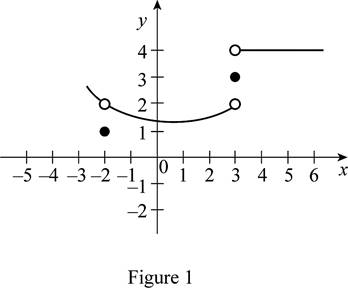# The graph of f ( x ) which satisfies the conditions, lim x → 3 + f ( x ) = 4 , lim x → 3 − f ( x ) = 2 , lim x → − 2 f ( x ) = 2 , f ( 3 ) = 3 and f ( − 2 ) = 1### Single Variable Calculus: Concepts...

4th Edition
James Stewart
Publisher: Cengage Learning
ISBN: 9781337687805### Single Variable Calculus: Concepts...

4th Edition
James Stewart
Publisher: Cengage Learning
ISBN: 9781337687805

#### Solutions

Chapter 2.2, Problem 15E
To determine

## To sketch: The graph of f(x) which satisfies the conditions,limx→3+f(x)=4,limx→3−f(x)=2,limx→−2f(x)=2, f(3)=3 and f(−2)=1

Expert Solution

### Explanation of Solution

Graph:

Given that, limx3+f(x)=4,limx3f(x)=2,limx2f(x)=2, f(3)=3 and f(2)=1.

Here, the left hand limit and the right hand limit of limx3f(x) are not the same.

That is, limx3+f(x)=4,limx3f(x)=2.

Hence, limx3f(x)=does not exist.

Moreover, according to the given conditions the graph must contain two points such that (3, 3) as f(3)=3 and (−2, 1) as f(2)=1.

Also observe that f(x) approaches 2 as x approaches −2.

Thus, the possible graph of f(x) is shown below in Figure 1.In Figure 1, open dot indicate that the points are excluded.

### Have a homework question?

Subscribe to bartleby learn! Ask subject matter experts 30 homework questions each month. Plus, you’ll have access to millions of step-by-step textbook answers!